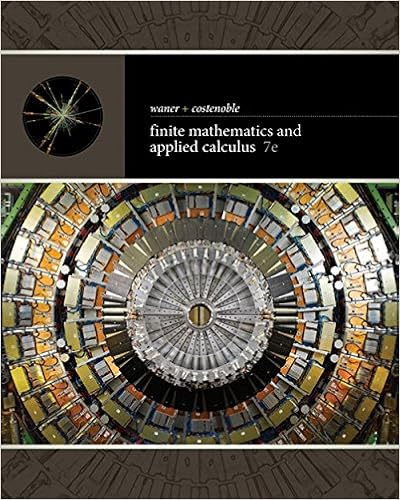# chapter 9.doc - Chapter 9 The Crisis 1 The Phillips curve...

• 9
• 100% (4) 4 out of 4 people found this document helpful

This preview shows page 1 - 3 out of 9 pages.

##### We have textbook solutions for you!
The document you are viewing contains questions related to this textbook.The document you are viewing contains questions related to this textbook.
Chapter 9 / Exercise 27
Finite Mathematics and Applied Calculus
Costenoble/WanerExpert Verified
Chapter 9: The Crisis1) The Phillips curve shows that when the unemployment rate is lower than the natural rate, A) inflation is higher than expected.B) inflation is lower than expected.C) policy rate is higher than expected.D) policy rate is lower than expected.Answer:
ADiff: 22) The Phillips curve shows that when the unemployment rate is higher than the natural rate,
BDiff: 23) Okun's law shows that when the unemployment rate is above the natural rate,
CDiff: 24) Okun's law shows that when the unemployment rate is below the natural rate,
DDiff: 25) Disposable income equalsA) income minus saving.B) income minus both saving and taxes.C) consumption minus taxes.D) the sum of consumption and saving.E) none of the aboveAnswer:
DDiff: 11
##### We have textbook solutions for you!
The document you are viewing contains questions related to this textbook.The document you are viewing contains questions related to this textbook.
Chapter 9 / Exercise 27
Finite Mathematics and Applied Calculus
Costenoble/WanerExpert Verified
6) In the IS-LM-PC model, which of the following is assumed to be exogenous?
ADiff: 27) In the IS-LM-PC model, investment does not depend on
ADiff: 28) In the IS-LM-PC model, LM curve is
ADiff: 29) The change in the unemployment rate is approximately equal toA) the negative of the growth rate of output.B) the negative policy rate.C) the negative inflation rate.D) the negative of the growth rate of money supply.Answer:
•••